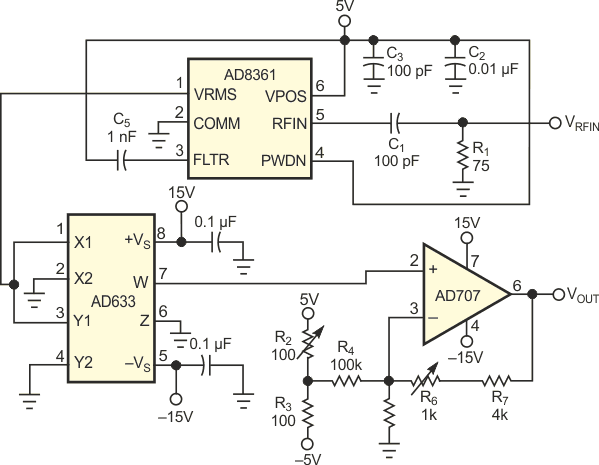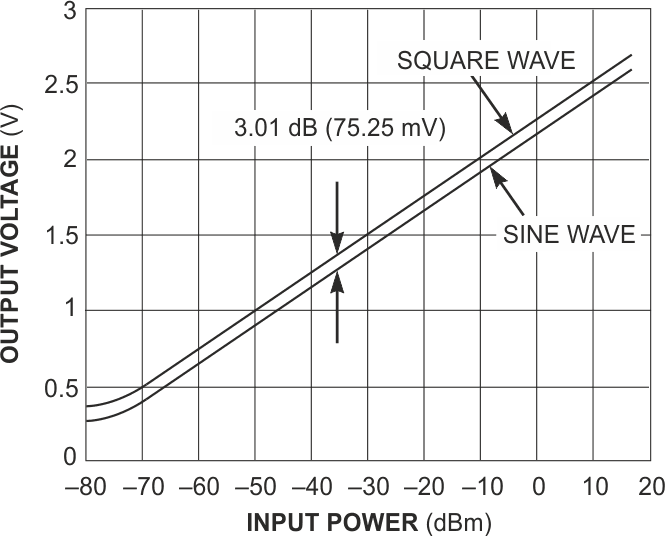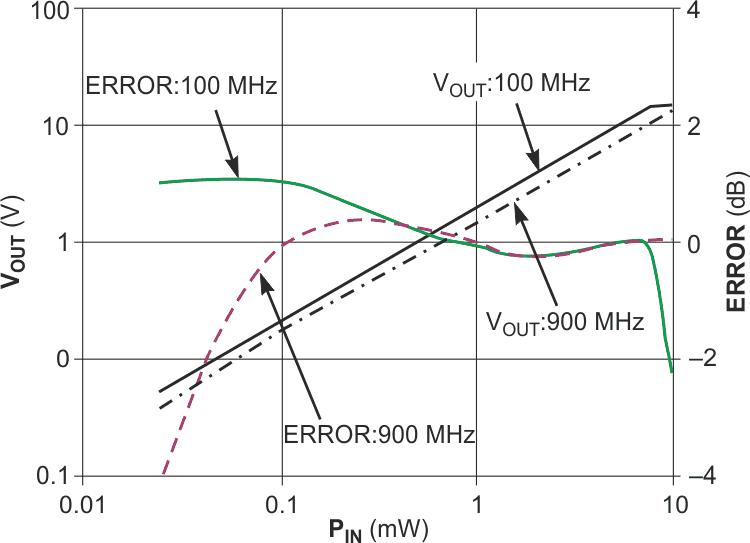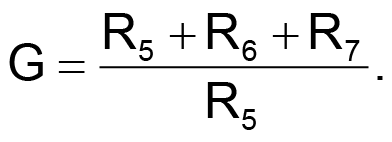# Make a truly linear RF-power detector

EDN

Modern high-performance transmitters require accurate monitoring of RF power, because most cellular standards depend on strict power- transmission levels to maintain an effective network. Regulation of transmitted-signal strength also lets you build lower cost systems. Figure 1 shows a waveform-independent circuit that provides a linear measurement of RF power. Sophisticated modulation schemes, such as CDMA (code-division multiple access) and TDMA (time-division multiple access) have obsoleted traditional approaches to RF power. Diode-based detectors have poor temperature stability, and thermal detectors have slow response times. Logarithmic amplifiers are temperature-stable and have a high dynamic range, but they exhibit a waveform-dependent response. This response causes the output to change with modulation type and, in the case of spread-spectrum technology, channel loading.Figure 1. This circuit provides an output voltage that is linearly proportional to the input power in watts.

Power detection must be waveform-independent in systems that use multiple modulation schemes. These include point-to-point systems that are configurable to transmit QPSK (quadrature-phase-shift keying), 16QAM (quadrature amplitude modulation), and 64QAM, for example, and spread-spectrum systems such as CDMA and W-CDMA (wide CDMA). A logarithmic amplifier in an automatic-gain-control loop can regulate the gain of a variable-gain power amplifier, but the output voltage is waveform-dependent, because the logarithmic amplifier does not respond to the rms level of the signal. For example, sine- and square-wave inputs that have the same rms voltage levels have different logarithmic intercepts (Figure 2). You could use calibration factors to correct this intercept difference in a multistandard system.Figure 2. Log amps detect signals over a wide dynamic range, but are not rms-responding.

An alternative solution (Figure 1) uses the AD8361, a high-frequency true-power detector. Unlike the logarithmic amplifier, the AD8361 is an rms-to-dc converter and, therefore, responds to the input rms voltage. Hence, a sine wave, a square wave, or any other input with the same rms level produces the same dc output, allowing you to incorporate waveform-independent measurement into a multimodulation system. With the addition of a multiplier, the circuit delivers an output voltage that is proportional to the input power level in watts. You can easily adjust gain and offset for this power meter with an op-amp circuit, thus providing an output scaled in volts per watt. A complex RF waveform feeds the input of the AD8361.Figure 3. The circuit in Figure 1 responds to rms signals, independent of waveform.

The multiplier squares the dc output to produce a voltage proportional to the power dissipated in the 50 Ω input impedance of the circuit. The AD633 multiplier squares the rms output of the AD8361 and divides by 10. The AD707 provides a maximum gain of 6:This value is lower than the gain of 10 that you would need to exactly cancel the effect of the multiplier scaling and allows the circuit to have a wider dynamic range, because the output would saturate with a smaller input with a gain of 10. You can easily adjust all circuit offsets with potentiometer R2. Figure 3 shows measurements made with this power meter. The graphs plot the output voltage and error for input signals at frequencies of 100 and 900 MHz. The detector operates at frequencies as high as 2.7 GHz.

EDN